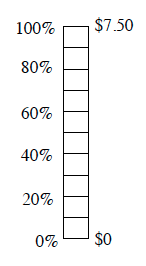### Home > CC2 > Chapter 5 > Lesson 5.3.2 > Problem5-111

5-111.

The local theater is raising ticket prices by $20\%$.

1. Assuming the current youth ticket price is $\7.50$, use the diagram at right to find out how much more youth tickets will cost.

First fill in the rest of the percent ruler. How much money does one space represent?

Each space represents $\0.75$. How much is $20\%$ if it covers two spaces?

The tickets will be $\1.50$ more.

2. What will be the new youth ticket price?